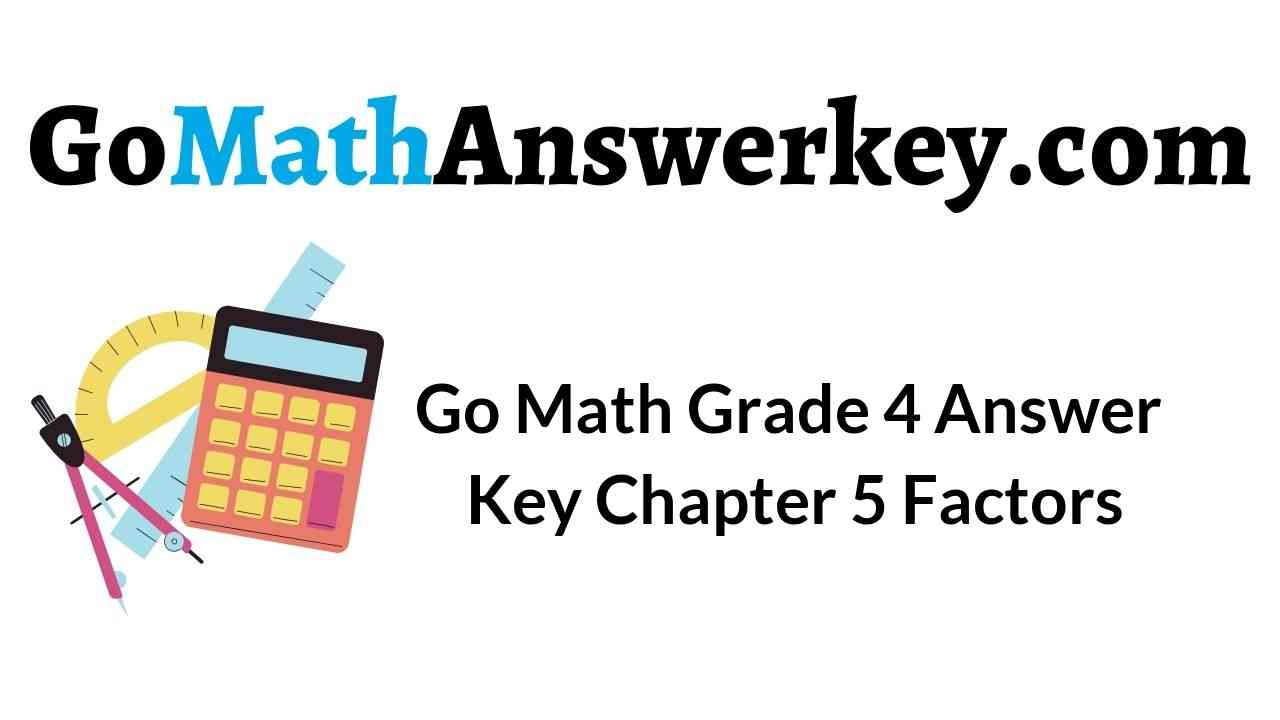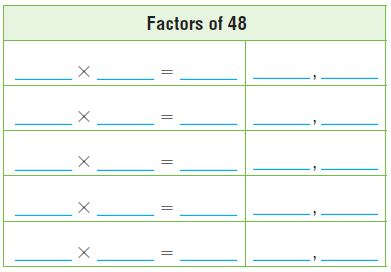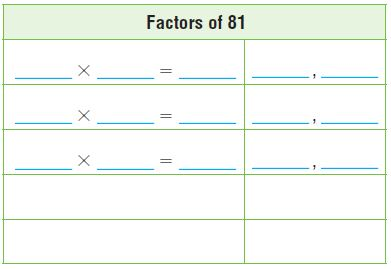# Go Math Grade 4 Answer Key Homework FL Chapter 5 Factors, Multiples, and Patterns Review/Test

Imporve your math skills by referring to the Go Math Grade 4 Answer Key Homework FL Chapter 5 Factors, Multiples, and Patterns Review/Test. With the support of this HMH Go Math Grade 4 Review/Test Answer Key you score good marks in the exam.

### Go Math Grade 4 Answer Key Homework FL Chapter 5 Factors, Multiples, and Patterns Review/Test

Go Math Grade 4 Answer Key Homework FL Review/Test covers all the topics in Chapter 5 Factors, Multiples, and Patterns. Explore the knowledge of your child by giving the question from Review/Test. Just hit on the link and download it. Go Math Grade 4 Answer Key Homework FL Chapter 5 Factors, Multiples, and Patterns Review/Test.

Chapter 5: Review/Test

### Review/Test – Page No. 219

Choose the best term from the box.Question 1.
The product of two numbers is a _______________ of both numbers.

The product of two numbers is a multiple of both numbers.

Question 2.
A _______________ has exactly two factors.

A prime has exactly two factors.

Question 3.
A number is always a multiple of its ____________ .

A number is always a multiple of its multiple.

List all the factor pairs in the table.

Question 4.Factors of 48 are 1,2,3,4,6.

Explanation:
1×48= 48    1,48.
2×24= 48    2,24.
3×16= 48     3,16.
4×12= 48     4,12.
6×8= 48       6,8

Question 5.Factors of 81 are 1,3,9.

Explanation:
1×81= 81     1,81
3×27= 81     3,27
9×9= 81       9,9

Is the number a multiple of 9? Write yes or no.

Question 6.
3 _____

Explanation:
The number 3 is a factor of 9 but not a multiple of 9.

Question 7.
39 _____

Explanation:
The number 39 is not a multiple of 39.

Question 8.
45 _____

Explanation:
9×5= 45, so the number 45 is a multiple of 9.

Question 9.
93 _____

Explanation:
The number 93 is not a multiple of 9.

Tell whether the number is prime or composite.

Question 10.
65 _________

Explanation:
As the number 65 factors are 1,5,13,65. So the number 65 is a composite number as it has more than two factors.

Question 11.
37 _________

Explanation:
The number 37 has only two factors 1 and 37, so the number is a prime number.

Question 12.
77 _________

Explanation:
The factors of 77 are 1,7,11 and 77, so the number 77 is a composite number.

Use the rule to write the first twelve terms in the pattern.
Describe another pattern in the numbers.

Question 13.

1,6,11,16,21,26,31,36,41,46,51,56.

Explanation:
1
(1+10)-5= 11-5= 6
(6+10)-5= 16-5= 11
(11+10)-5= 21-5= 16
(16+10)-5= 26-5= 21
(21+10)-5= 31-5= 26
(26+10)-5= 36-5= 31
(31+10)-5= 41-5= 36
(36+10)-5= 46-5= 41
(41+10)-5= 51-5= 46
(46+10)-5= 56-5= 51
(51+10)-5= 61-5= 56.

### Review/Test – Page No. 220

Question 14.
Erica knits 18 squares on Monday. She knits 7 more squares each day for the rest of the week. How many squares does Erica have on Friday?
Options:
a. 36
b. 46
c. 54
d. 90

Explanation:
As Erica knits 18 squares on Monday and she knits 7 more squares each day for the rest of the week, so on Friday Erica have 18+7+7+7+7= 46.

Question 15.
James works in a flower shop. He will put 36 tulips in vases for a wedding. He must use the same number of tulips in each vase. How many tulips could be in each vase?
Options:
a. 1, 2, 8
b. 2, 4, 8
c. 2, 4, 9
d. 6, 12, 16

Explanation:
As James put 36 tulips in vases for a wedding and he must use the same number of tulips in each vase, so we must find the factors of 36 to find how many tulips could be in each vase. So the factors of 36 are 1,2,3,4,6,9,12,18,36. So
2 tulips in 18 vases each
4 tulips in 9 vases each
9 tulips in 4 vases each.

Question 16.
What multiple of 7 is a factor of 7?
Options:
a. 0
b. 1
c. 7
d. 14

Explanation:
The number 7 is multiple and a factor of 7.

Question 17.
Hot dogs come in packages of 6. Hot dog buns come in packages of 8. Antonio will buy the same number of hot dogs as hot dog buns. How many hot dogs could he buy?
Options:
a. 6
b. 8
c. 18
d. 24

Explanation:
As hot dogs come in packages of 6, and hot dog buns come in packages of 8. So to find how many hot dogs could Antonio bought we must find the multiples of 6 and 8. So multiples of 6 and 8 are
Multiples of 6 are 6, 12, 18, 24, 30
Multiples of 8 are 8, 16, 24, 32, 40.
So Antonio bought 24 hot dogs.

Question 18.
Sean has 54 flower bulbs. He planted all the bulbs in rows. Each row has the same number of bulbs. How many bulbs could be in each row?
Options:
a. 6
b. 8
c. 12
d. 26

Explanation:
As Sean has 54 flower bulbs and planted all the bulbs in rows and each row has the same number of bulbs, so we will find the factors of 54. And the factors of 54 are 1,2,3,6,9,18,27, and 54. So Sean will plant 6 bulbs in each row.

### Review/Test – Page No. 221

Question 19.
An ice-cream truck visits Julio’s street every 3 days and Lara’s street every 4 days. The truck visits both streets on April 12. When will the truck visit both streets next?
Options:
a. April 15
b. April 16
c. April 19
d. April 24

Explanation:
As an ice-cream truck visits Julio’s street every 3 days and Lara’s street every 4 days, and the truck visits both streets on April 12, so the next visit will be on April 24. By finding the multiples of 3 and 4 we will get the answer.
Multiples of 3 are 3,6,9,12,15,18,21,24
Multiples of 6 are 6,12,18,24.

Question 20.
The factors of a number include 2, 3, 4, 6, 8, 12, 16, 32, and 48. Which could be the number?
Options:
a. 32
b. 64
c. 96
d. 98

Explanation:
As the number 96 is divisible by all the given numbers.

Question 21.
Ms. Booth has 16 red buttons and 24 blue buttons. She is making finger puppets. Each puppet has the same number of blue buttons and red buttons. How many puppets can she make if she uses all of the buttons?
Options:
a. 1, 2, 4, or 8
b. 1, 2, 4, 8, or 16
c. 1, 2, 4, 8, or 24
d. 1, 2, 4, 8, 16, or 24

Explanation:
As Ms. Booth has 16 red buttons and 24 blue buttons and she is making finger puppets and each puppet has the same number of blue buttons and red buttons, so to find how many puppets can she make if she uses all of the buttons we will find the factors of 16 and 24
so the factors of 16 are 1,2,4,8,16
Factors of 24 are 1,2,3,4,6,8,12,24.
So the common factors in both 16 and 24 are 1,2,4,8,16.

### Review/Test – Page No. 222

Question 22.
I am a number between 60 and 100. My ones digit is two less than my tens digit. I am a prime number. What number am I?
Explain.
_____

Explanation:
Let’s name the digit:
X be one’s digit and y be tens digit
we know that X=Y-2. Now, Y can be 6,7,8,9 the number is between 60 and 100
As the possibilities with x=y-2, the numbers would be 64,75,86,97.
And 64 and 86 are even, so they can’t be prime. 75 is a composite number as there are more than two factors. So the remaining number is 97.

Question 23.
The number of pieces on display at an art museum is shown in the table.A. The museum’s show for July features 30 oil paintings by different artists. All artists show the same number of paintings and each artist shows more than 1 painting. How many artists could be featured in the show?

15 artists with 2 paintings per artist.
10 artists with 3 paintings per artist.
6 artists with 5 paintings per artist.
5 artists with 6 paintings per artist.
3 artists with 10 paintings per artist.
2 artists with 15 paintings per artist.

Explanation:
As the museum’s show for July features 30 oil paintings by different artists and all artists show the same number of paintings and each artist shows more than 1 painting, so the number of artists are
15 artists with 2 paintings per artist.
10 artists with 3 paintings per artist.
6 artists with 5 paintings per artist.
5 artists with 6 paintings per artist.
3 artists with 10 paintings per artist.
2 artists with 15 paintings per artist.

Question 23.
B. The museum wants to display all the art pieces in rows. Each row has the same number of pieces and the same type of pieces. How many pieces could be in each row?

Explanation:
Given that 30 oil paintings, 24 photographs, and 21 sketches that a museum wants a display. The arrangement of all these art pieces must be in rows such that each row has the same number and same type of art piece displayed. And the greatest common factor of 30,24,21 is 3. So 3 pieces could be in each row.

Question 23.
C. The museum alternates between adding 3 new pieces one month and retiring one piece the following month. If the museum starts with 75 pieces and the pattern continues, write the numbers in the pattern for the next 8 months. Describe other patterns in the numbers.

Answer: 78, 77, 80, 79, 82, 81, 84, 83.

Explanation:
As the museum alternates between adding 3 new pieces one month and retiring one piece the following month and if the museum starts with 75 pieces and the pattern continues, so the numbers are 78, 77, 80, 79, 82, 81, 84, 83. Here the pattern is every other number differs by 2 and the numbers alternate between even and odd.

Conclusion:

The scholars of 4th grade can check all chapters in Go Math Grade Answer Key in pdf format so that your learning will kick start in an effective manner. We have given a brief explanation of each and every question on our Go Math Grade 4 Answer Key Homework FL Chapter 5 Factors, Multiples, and Patterns Review/Test. We recommend the students to understand the concepts and apply them in the real world.

Scroll to Top